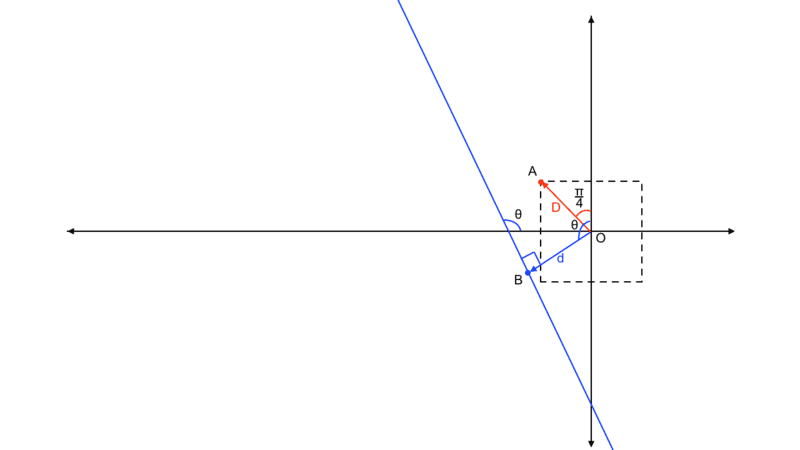# What is the best shape for a soccer goal post?

Homework Helper
For smaller angles of ##\theta## the ball would strike below the point D so how can the ball still go in?
The initial strike on the left hand pole was below the due-east point on that pole. Yet you busy are calculating how that strike can go in.

Now you have a rebound that strikes the right hand pole below its due-west point. There is still calculation left to do.

Staff Emeritus
Gold Member
In reality, shots that touch the inner part of the post are more likely than shots that touch the outer part because the player is attempting to get it in the goal.

This is making an assumption about the accuracy of shots. My understanding is a significant fraction of shots don't even hit the net, so I would guess the 1" difference between the inner and outer part of the post is pretty insignificant for the probability distribution.

Homework Helper
Gold Member
2022 Award
This is making an assumption about the accuracy of shots.
No, only that the distribution is unimodal, but I did not state it correctly. I meant that of the parts of the post the player can see, there will be a bias towards the side nearer the goal mouth. Anyway, I agree it can probably be ignored.
Far subtler is the distribution of where shots are made from. Obviously the attacker would like to make the shot from in front of the goal, and the closer to the centreline the better; but the throng of defenders would lead to less ideal choices.

Homework Helper
Gold Member
2022 Award
I'll need a figure to understand that but right now I have to go, maybe I'll come back to that later.

Please see my edit to post #68.

kshitij
It is easiest to see it by running it in reverse.
Suppose the ball eventually hits the half way around point of one of the posts, but does so approaching from almost parallel to the goal line, making some tiny angle ##\alpha## to it.
Trace that back to its last bounce off the other post, and back again towards the first, and so on until it misses a post. Clearly, by making ##\alpha## small enough there is no limit to the number of bounces.
The algebra will be messy...
Edit: it goes like this. I'll describe it as though the ball starts at the halfway around point of one post and progressively bounces out of the goal.
Let the posts be 1, 2 and the distance between them along their line of centres, L, be D>>R.
From 1, it hits 2 roughly ##D\alpha## from L, at a point subtending angle ##\frac DR\alpha## to that line at the centre of 2.
It bounces from there at an angle ##(1+2\frac DR)\alpha## to L, do you see that?
Can you generalise it to n bounces?
So, I think this is the situation you are talking about (on decreasing the angle between the line joining the centres and path of the ball (red), number of bounces increases),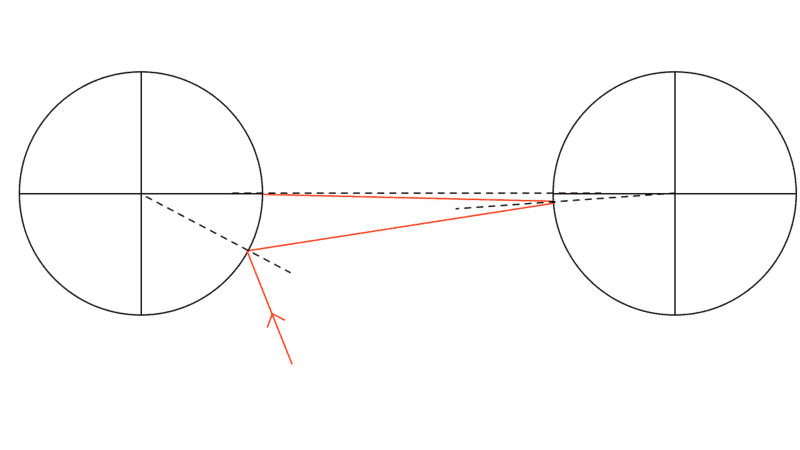This infact, does contradict what I thought earlier the situation would be for a ball striking the far post below the midpoint,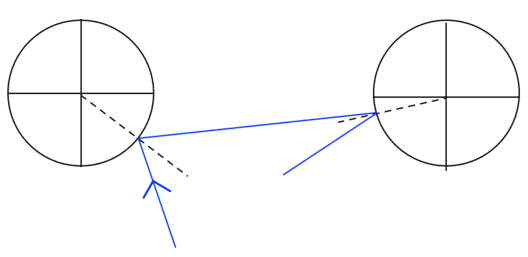But on closer inspection, I spotted a difference between the two,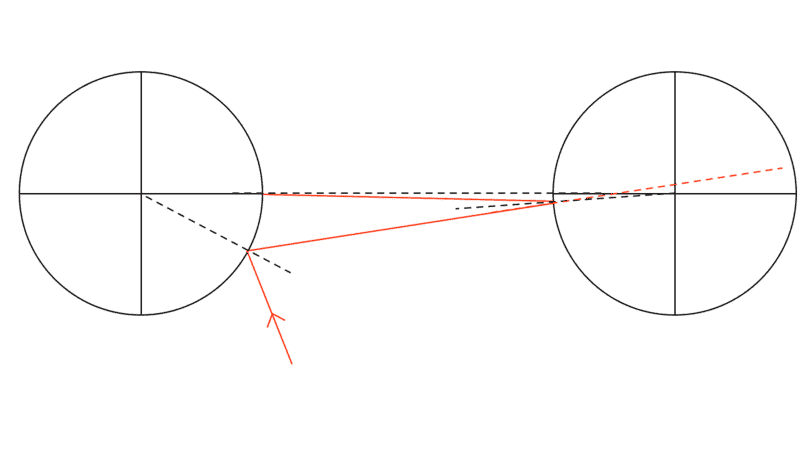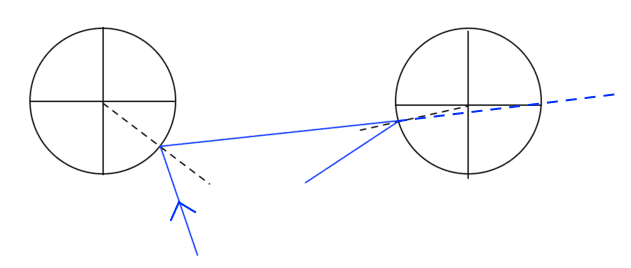We can quite clearly see that on extending the path of the ball, the red one passes above the centre, while the blue one passes below the centre.

So, I speculate this (below) might be the correct limiting case (feel free to correct me),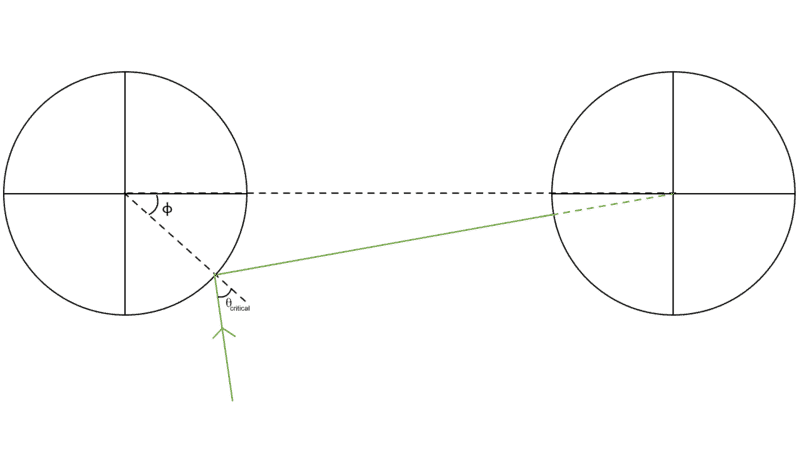Here the path passes through the centre of the far post and on rebounding off the far post, the path of the ball retraces itself.

Am I getting it right this time?

#### Attachments

Last edited:
kshitij
So, I speculate this (below) might be the correct limiting case (feel free to correct me),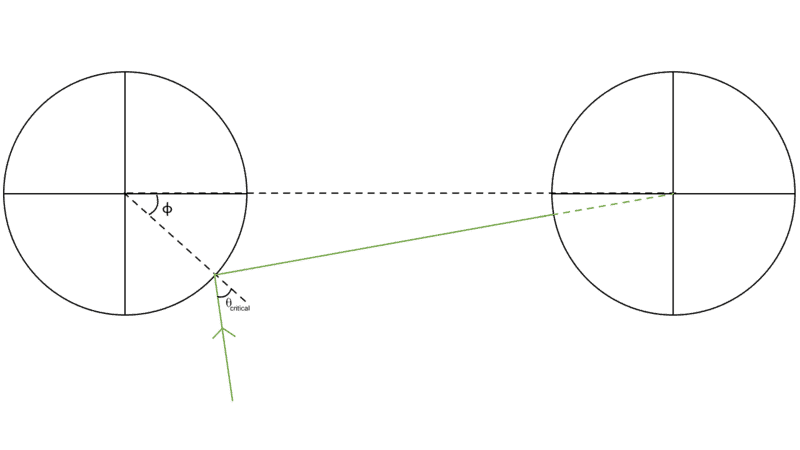Now I don't think that there is any smaller value of ##\theta## possible for this given ##\phi## because anything smaller than this will bounce away from the goal side after hitting the far post. So, I think that if we do what @haruspex said,
Edit: it goes like this. I'll describe it as though the ball starts at the halfway around point of one post and progressively bounces out of the goal.
Let the posts be 1, 2 and the distance between them along their line of centres, L, be D>>R.
From 1, it hits 2 roughly Dα from L, at a point subtending angle DRα to that line at the centre of 2.
It bounces from there at an angle (1+2DR)α to L, do you see that?
Can you generalise it to n bounces?
And generalise that to n bounces, (according to what I think) the (n-1)th (*penultimate) bounce will be when the extended path of the ball just passes through the centre of either of the posts. So, we both should get the same result through both the methods. Doing the reverse process though will be much more complicated, so I think that solving the above critical case is the way to do it.

(*by penultimate bounce what I mean is that, we cannot keep on bouncing back and forth between the posts forever, there must be a time when the ball rebounding off one post will not bounce back onto the other post, thus the second last bounce is the penultimate bounce which you can picture by simply reversing the green path in the above image)

Edit: I do realize that if D>>R then there will be no limit on the number of bounces, but D=7.32m & R=0.06m, so I think we can't say that D>>R

Last edited:
Homework Helper
Gold Member
2022 Award
So, I think this is the situation you are talking about (on decreasing the angle between the line joining the centres and path of the ball (red), number of bounces increases),
View attachment 282741
This infact, does contradict what I thought earlier the situation would be for a ball striking the far post below the midpoint,
View attachment 282747
But on closer inspection, I spotted a difference between the two,
View attachment 282743View attachment 282744
We can quite clearly see that on extending the path of the ball, the red one passes above the centre, while the blue one passes below the centre.

So, I speculate this (below) might be the correct limiting case (feel free to correct me),
View attachment 282745
Here the path passes through the centre of the far post and on rebounding off the far post, the path of the ball retraces itself.

Am I getting it right this time?
You've gone a bit too far the other way. You could increase theta a little and still not score a goal.
E.g. if after the second bounce it travels parallel to the line of centres then after the third it will be moving away from the goal line.
It needs to keep getting a bit closer to the goal line each bounce. In fact, the angle to the goal line must reduce by a factor a bit greater than 2 (about 2+R/D.)

•kshitij and jbriggs444
Homework Helper
Gold Member
2022 Award
I do realize that if D>>R then there will be no limit on the number of bounces, but D=7.32m & R=0.06m, so I think we can't say that D>>R

It is always true that there is no limit on the number of bounces. Running it backwards, the smaller you make the initial angle to the goal line the more bounces it will take to escape.

You don't think D>100R constitutes D>>R?

•kshitij
kshitij
You've gone a bit too far the other way. You could increase theta a little and still not score a goal.
E.g. if after the second bounce it travels parallel to the line of centres then after the third it will be moving away from the goal line.
I did try to visualise that and it seems correct.
It is always true that there is no limit on the number of bounces. Running it backwards, the smaller you make the initial angle to the goal line the more bounces it will take to escape.

You don't think D>100R constitutes D>>R?
Yes, I think you are right here.

Jan Jaroscak
@kshitij Did you manage to find any solution to the problem? I am trying to explore a similar question and I would probably make the same assumptions as you (even if that didn't lead to an answer that is representative of reality). I agree that the probability of the ball going in off a square post is 33% and 25%+ for the circular post, but I do not know how to figure out how much more than 25% it is.

Staff Emeritus
Homework Helper
Gold Member
@kshitij Did you manage to find any solution to the problem? I am trying to explore a similar question and I would probably make the same assumptions as you (even if that didn't lead to an answer that is representative of reality). I agree that the probability of the ball going in off a square post is 33% and 25%+ for the circular post, but I do not know how to figure out how much more than 25% it is.
Again, these assumptions are faulty even if the ball is infinitesimal in size. The answer must depend on the distribution of incoming balls.

Jan Jaroscak
Again, these assumptions are faulty even if the ball is infinitesimal in size. The answer must depend on the distribution of incoming balls.
The distribution of incoming balls is not relevant for the question that I (and from what I understand @kshitij as well) am asking, although it may be more representative of reality. My question is simply: Which goal post allows for a bigger angle for a goal to be scored after reflection – square or circular? And of course, find the exact critical angle. The image below explains why I believe the square post allows for a 90-degree angle resulting in a goal while the circular post allows for π/2 + θ. θ is what I am trying to find.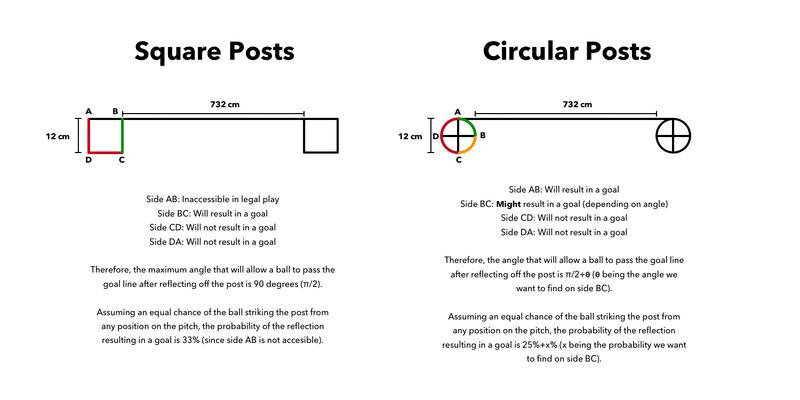•kshitij
Homework Helper
Gold Member
2022 Award
The distribution of incoming balls is not relevant for the question that I (and from what I understand @kshitij as well) am asking,
In order to get an answer at all you have to assume a distribution. You must be doing that even if you don't realize.
There are two aspects: the direction of the ball and the lateral displacement. You could take a uniform distribution across the legal range for each independently. For a square post, that gives a 1/4 chance of a goal, not 1/3.
For round posts, in your diagram, the chance of hitting arc AB wth that distribution is (I think) ##\frac 14-\frac 1{2\pi}##.
As noted in post #50 onwards, finding the critical angle/displacement in BC for round posts is quite tricky because there can be an unlimited number of rebounds between the posts before the outcome is clear. The distance between the posts is relevant.

Last edited:
•Jan Jaroscak and Orodruin
Homework Helper
Gold Member
2022 Award
The distribution of incoming balls is not relevant for the question ...
You could consider only penalty kicks, for example. Otherwise, where is the ball being projected from?

•kshitij
Jan Jaroscak
You could consider only penalty kicks, for example. Otherwise, where is the ball being projected from?
That is actually a great idea! Instead of having to consider both the direction of the ball and the point of impact as varying factors like before, it is now possible to combine the two since the starting position will always be the same. In that case, am I correct in saying that the ball will end up in the goal when it reflects off the circular post anywhere to the right of the red ray in the diagram below? An example of this is the green ray. A ball (still assuming it is a point mass) traveling along the red ray would simply reflect in the same direction it came from as the ray is parallel to the normal. Any collision left of the red ray would cause the ball to reflect away from the goal line.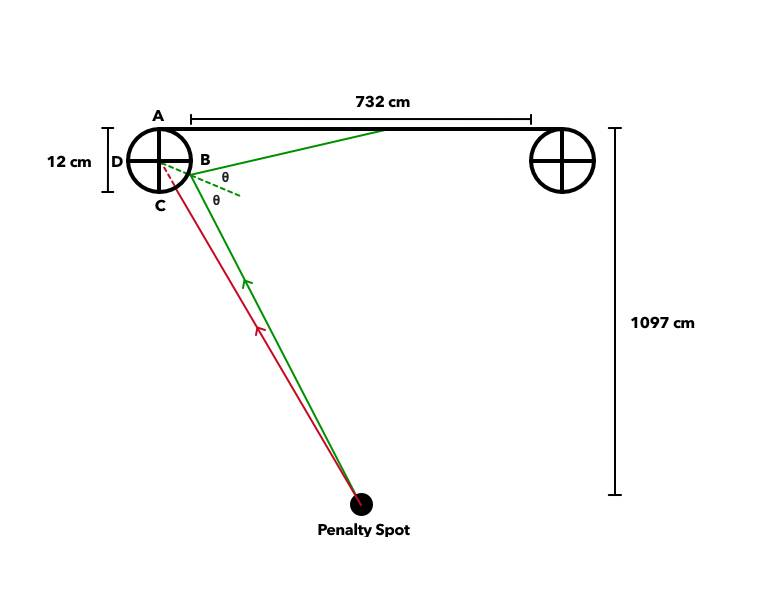#### Attachments

Staff Emeritus
Homework Helper
Gold Member
You could consider only penalty kicks, for example. Otherwise, where is the ball being projected from?
Even that is not exactly representative of reality because of curve balls.

Homework Helper
Gold Member
2022 Award
Even that is not exactly representative of reality because of curve balls.
Curve balls are in baseball, surely? We used to call them banana shots!

•Jan Jaroscak and fresh_42
Jan Jaroscak
Right, but assuming the ball is a point mass, the interaction between the post and the ball would be a definite point, rather than an area, resulting in the reflected ray being straight. Am I missing something here?
Even that is not exactly representative of reality because of curve balls.

Homework Helper
Right, but assuming the ball is a point mass, the interaction between the post and the ball would be a definite point, rather than an area, resulting in the reflected ray being straight. Am I missing something here?
The concern was estimating the distribution of shot angles and/or displacements. One possible way to arrive at such a distribution would be to start with a distribution of points from which a shot was attempted.

But if you fail to account for "bananas"/"curve balls" then this will not lead to a correct distribution of arrival angles.

In addition, the reflected rays need not be straight if the balls rebound from a post with non-negliglble spin.

[It is not clear whether the idealization of the ball as a point mass is intended to do away with Magnus lift or to retain and idealize it as well]

•Orodruin
Homework Helper
Gold Member
2022 Award
It seems to me now that the wrong question is being asked. The probability of a goal depends also on the thickness of the posts.
If we say circular posts are radius R and square posts are of side 2S then the question becomes what the ratio of R to S should be to make them equal.
To evaluate that, we need to consider the range of displacement of the trajectory from the centre line (the to the centre of the post) to be ##\pm D## where ##D>\max\{R,S\sqrt 2\}##.

Homework Helper
Gold Member
2022 Award
It seems to me now that the wrong question is being asked. The probability of a goal depends also on the thickness of the posts.
If we say circular posts are radius R and square posts are of side 2S then the question becomes what the ratio of R to S should be to make them equal.
To evaluate that, we need to consider the range of displacement of the trajectory from the centre line (the to the centre of the post) to be ##\pm D## where ##D>\max\{R,S\sqrt 2\}##.
Continuing that line ...
The centre of a post is at the origin. If square, the post has corners at ##(\pm S, \pm S)##. If circular , it is radius R.
The other post is at ##(-\infty,0)##, to avoid worrying about rebounds off both posts.
The ball approaches along a line making angle ##\theta## to the positive x axis. Its line would pass distance d to the right of the origin.
The angle is uniformly distributed in ##(0,\pi)##. d is uniformly distributed in (-D,D) where ##D>R ## and ## D>S\sqrt 2##.
1. Square post.
A goal is scored if and only if the ball passes to the right of ##(-D,D)##. That is, if ##d>S\sqrt 2\cos(\frac{\pi}4-\theta)##. The probability of a goal is therefore ##\frac 1{2D\pi}\int_0^\pi D-S\sqrt 2\cos(\frac{\pi}4-\theta)d\theta##.
2. Round post
Certainly it is a goal if d>R.
Suppose the ball strikes the post at ##(R,\theta+\alpha)## in polar coordinates. So ##d=R\sin(\alpha)##.
It will rebound at angle ##\theta+2\alpha## to the x axis. A goal will result if that exceeds ##\pi##, i.e. ##d>R\cos(\frac \theta 2)##.
The probability of a goal is therefore ##\frac 1{2D\pi}\int_0^\pi(D-R\cos(\frac\theta 2)).d\theta##.

For what relative R and S are those equal? I get R=S(!)
If we consider wide angle shots rare, we could take the integration range as ##(\pi/4,3\pi/4)##, say, giving ##S=2\sin(\pi/8)R##.

Last edited:
•kshitij
Homework Helper
2022 Award
To evaluate that, we need to consider the range of displacement of the trajectory from the centre line (the to the centre of the post) to be ±D where D>max{R,S2}.
I agree but if you track the position of the center of the ball you need to include the finite radius of the ball ##\equiv R_{ball}##. In each case the effective shape is obtained by "moving" the ball over the post and tracking the center. For the circular post the effective radius just becomes ##R+R_{ball}##. For the square post it will be more complicated: a rounded corner (radius ##R_{ball}##) square post.

Edit: Sorry I missed your earlier posts (#24et seq?) It seems a very bad approximation right at the heart of the issue...

Last edited:
Homework Helper
Gold Member
2022 Award
I agree but if you track the position of the center of the ball you need to include the finite radius of the ball ##\equiv R_{ball}##. In each case the effective shape is obtained by "moving" the ball over the post and tracking the center. For the circular post the effective radius just becomes ##R+R_{ball}##. For the square post it will be more complicated: a rounded corner (radius ##R_{ball}##) square post.

Edit: Sorry I missed your earlier posts (#24et seq?) It seems a very bad approximation right at the heart of the issue...
Not sure what you mean by the last sentence.
As I noted in post #28, although the ball is rather fatter than the post, for the purposes of comparing different post shapes it is probably ok to treat the ball as a point.

kshitij
The probability of a goal depends also on the thickness of the posts.
Yes, but the thickness of posts are fixed, I wanted to know the probabilities for only the cases when ##L=2R## where ##L## is the side length of square post and ##R## is the radius of circular post.
To evaluate that, we need to consider the range of displacement of the trajectory from the centre line (the to the centre of the post) to be ±D where D>max{R,S2}.
As I understand if ##D>\max\{R,S\sqrt 2\}## then why would the ball even hit the posts? Yes it would go in but I wanted to know the probability of a shot going in after it hits the post.
A goal is scored if and only if the ball passes to the right of (−D,D). That is, if d>S2cos⁡(π4−θ).
I don't understand why you multiplied ##S\sqrt{2}## with ##\cos(\frac{\pi}{4}-\theta)##? Maybe a figure would help? If the ball passes to the right of ##(-D,D)## which is marked as point ##A## in the figure and I think that you assumed the other post to be at ##(-\infty,0)## then how will the ball ever cross the line?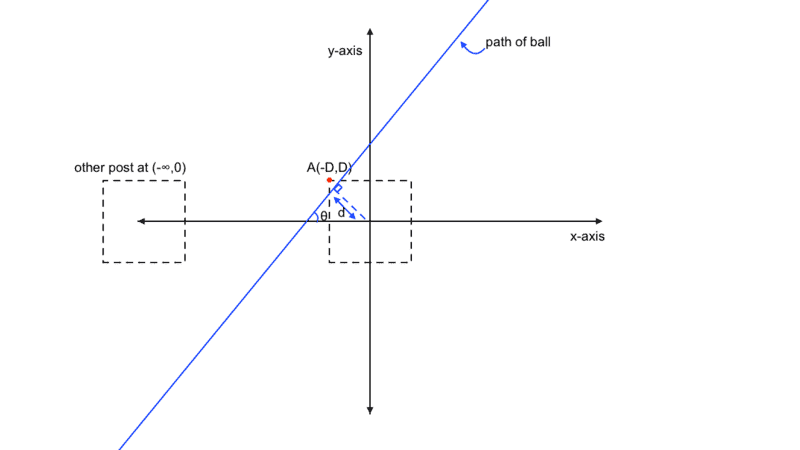For what relative R and S are those equal? I get R=S(!)
I didn't understand how you got this but are you saying that both posts have equal probabilities? If yes then this was exactly what I wanted! but I am a bit surprised that the probabilities are equal

kshitij
That is actually a great idea! Instead of having to consider both the direction of the ball and the point of impact as varying factors like before, it is now possible to combine the two since the starting position will always be the same. In that case, am I correct in saying that the ball will end up in the goal when it reflects off the circular post anywhere to the right of the red ray in the diagram below? An example of this is the green ray. A ball (still assuming it is a point mass) traveling along the red ray would simply reflect in the same direction it came from as the ray is parallel to the normal. Any collision left of the red ray would cause the ball to reflect away from the goal line.
View attachment 287605
The red ray is definitely not the limiting case! the limiting case should be when the green line is tangent to the other post for no rebound by the second post and for only one rebound by the second post the green line should end up in the top left quarter of the second post.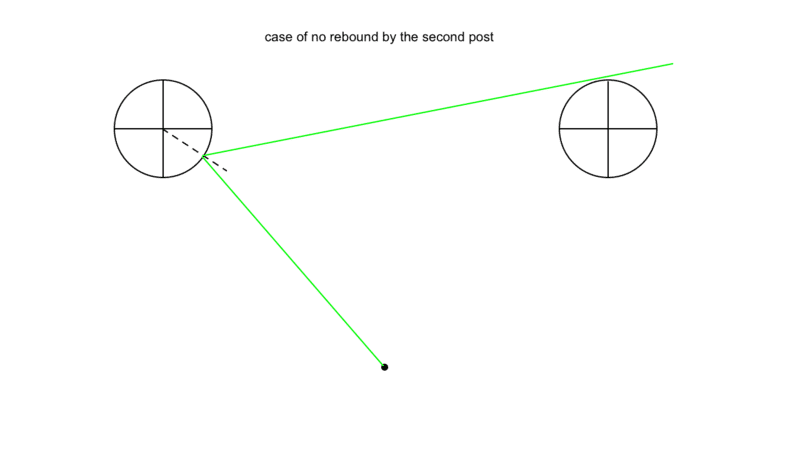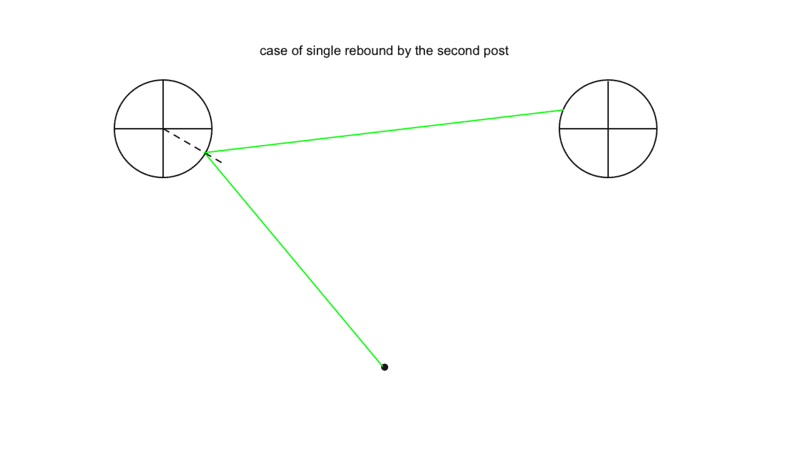kshitij
I am again saying that the whole point of the post was to find which of the two posts are better under similar situations (which may not be accurate representation of reality) because I wondered why did they replace the square ones with circular or elliptical ones? does really (under some specific conditions) the round post leads to more shots going in that the square one? or maybe it was just because they thought that the square ones are more dangerous for players?

Homework Helper
Gold Member
2022 Award
I wanted to know the probabilities for only the cases when L=2R where L is the side length of square post and R is the radius of circular post.
if D>max{R,S2} then why would the ball even hit the posts?
To make a proper comparison, you must not only consider the cases where the ball hits the post. There will be trajectories that would hit the one post shape but not the other. So we must set D large enough to encompass both posts.
If the ball passes to the right
That's to the right from the ball's perspective, so to the left in the diagram.
I didn't understand how you got this but are you saying that both posts have equal probabilities? If yes then this was exactly what I wanted! but I am a bit surprised that the probabilities are equal
For the distribution of trajectories I chose and equal widths of posts (L=2S=2R), yes - I was surprised too. But as noted, if you change the distribution the answer changes.

kshitij
To make a proper comparison, you must not only consider the cases where the ball hits the post. There will be trajectories that would hit the one post shape but not the other. So we must set D large enough to encompass both posts.

That's to the right from the ball's perspective, so to the left in the diagram.

For the distribution of trajectories I chose and equal widths of posts (L=2S=2R), yes - I was surprised too. But as noted, if you change the distribution the answer changes.
And how did you get ##d>S\sqrt 2\cos(\frac{\pi}4-\theta)##?
Also I hope that this diagram is now correct,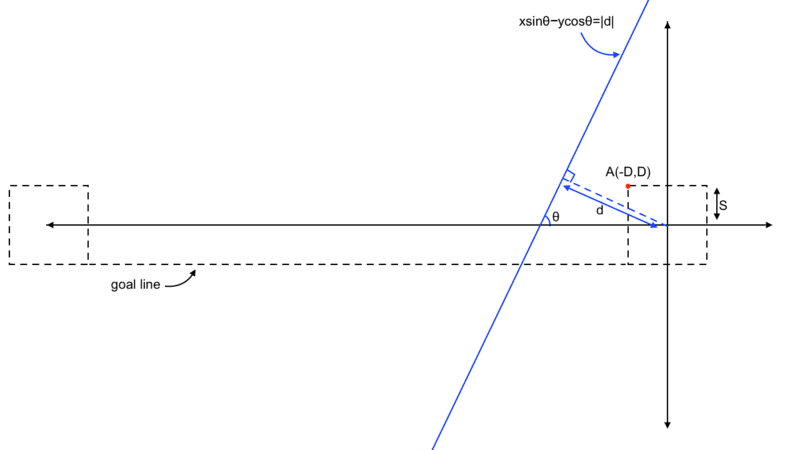Homework Helper
Gold Member
2022 Award
And how did you get ##d>S\sqrt 2\cos(\frac{\pi}4-\theta)##?
Also I hope that this diagram is now correct,
View attachment 287708
Draw the trajectory passing through A=(-D,D).
If its nearest point to the origin, O, is B, what is angle BOA?

kshitij
Draw the trajectory passing through A=(-D,D).
If its nearest point to the origin, O, is B, what is angle BOA?
Yes I get OB=##S\sqrt2\cos(\frac{\pi}{4}-\theta)##, next step?

kshitij
Yes I get OB=##S\sqrt2\cos(\frac{\pi}{4}-\theta)##, next step?
Are we just considering the cases where ##d \gt S\sqrt2\cos(\frac{\pi}{4}-\theta)## but this will not give the cases when the ball goes in after deflection from the post, this was not what I was asking

kshitij
Are we just considering the cases where ##d \gt S\sqrt2\cos(\frac{\pi}{4}-\theta)## but this will not give the cases when the ball goes in after deflection from the post, this was not what I was asking
I thought you calculated the probability of a shot going in after hitting the post, this was what I was originally asking, "of all the shots that hit the post which of the two posts (square or round) has a greater probability for those shots going in"

Homework Helper
Gold Member
2022 Award
this will not give the cases when the ball goes in after deflection from the post
It will. Draw the diagram for ##\theta>\pi/2## and d just sufficient to pass to the right of A.

kshitij
It will. Draw the diagram for ##\theta>\pi/2## and d just sufficient to pass to the right of A.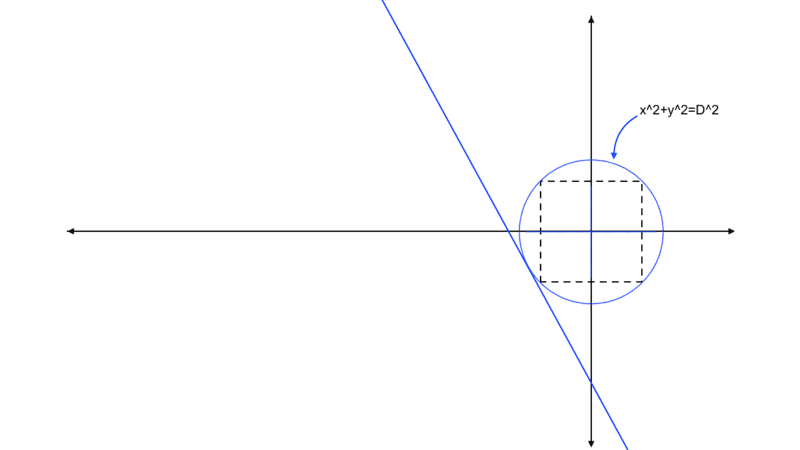The blue circle is of radius ##D## and if the path of the ball is tangent to that circle (because ##d## is the perpendicular distance of the path from origin and this distance is equal to ##D## in the limiting case) it will not hit the post

kshitij
View attachment 287709
The blue circle is of radius ##D## and if the path of the ball is tangent to that circle (because ##d## is the perpendicular distance of the path from origin and this distance is equal to ##D## in the limiting case) it will not hit the post
I realized you said that the limiting case is ##d=D\cos(\frac{\pi}{4}-\theta)## not ##d=D## but now I cannot understand why?
What I do understand is that ##\left|\frac{\pi}{4}-\theta\right|## is the angle between the vectors ##\vec {OA}## and ##\vec {OB}## where ##\vec {OB}## is the perpendicular distance from origin ##O## to the path of the ball and ##D\cos(\frac{\pi}{4}-\theta)## is the projection of ##\vec {OA}## on ##\vec {OB}##